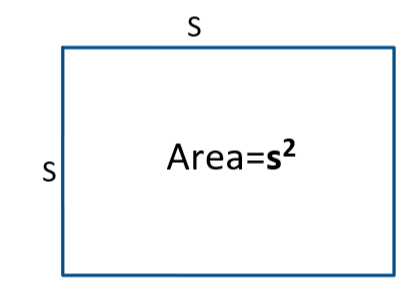Courses
Courses for Kids
Free study material
Offline Centres
More

# Mensuration Class 6 Notes CBSE Maths Chapter 10 (Free PDF Download)Last updated date: 28th Nov 2023
Total views: 589.2k
Views today: 14.89k

## Revision Notes for CBSE Class 6 Maths Chapter 10 - Free PDF Download

Free PDF download of Class 6 Maths Chapter 10 - Mensuration Revision Notes & Short Key-notes prepared by expert Maths teachers from latest edition of CBSE(NCERT) books. To register Maths Tuitions on Vedantu.com to clear your doubts.

## Access Class 6 Maths Chapter 10 - Mensuration Notes

• Perimeter is the total length of the boundary forming around a closed figure, going round the figure once.

1. Perimeter of a rectangle$\text{= 2 }\!\!\times\!\!\text{ }\left( \text{length + breadth} \right)\text{ }\!\!~\!\!\text{ }$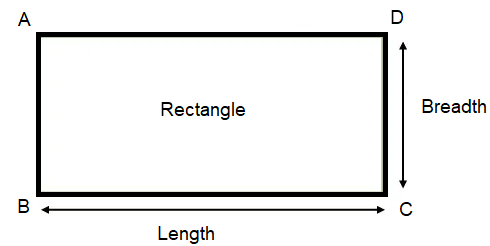1. Perimeter of a square $\text{= 4 }\!\!\times\!\!\text{ length of its side}$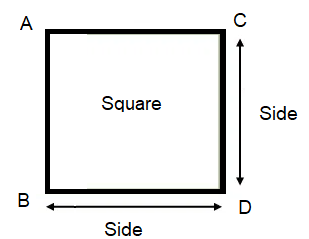1. Perimeter of an equilateral triangle $\text{= 3 }\!\!\times\!\!\text{ length of a side }\!\!~\!\!\text{ }\!\!~\!\!\text{ }\!\!~\!\!\text{ }\!\!~\!\!\text{ }\!\!~\!\!\text{ }$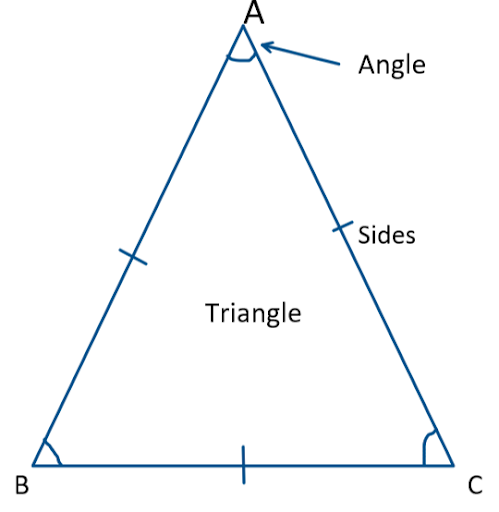1. Perimeter of a regular pentagon  $\text{= 5 }\!\!\times\!\!\text{ length of a sides}$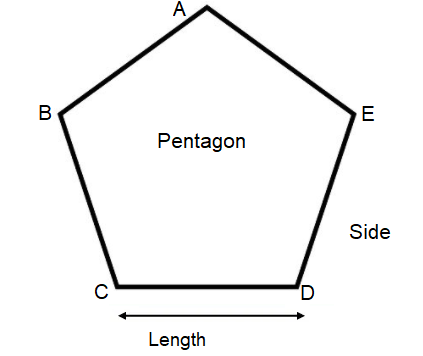• Regular closed figures are those with the same number of sides and angles on all sides.

• The area of a closed figure refers to the amount of surface it covers.

• The following conventions are used to compute the area of a figure on squared paper:

1. Parts of the area that are less than half a square should be ignored.

2. If a region contains more than half a square. It should be counted as one square.

3. Take the size of the square in sq units if exactly half of it is counted.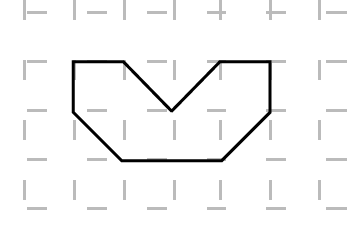Here to make this closed figure, we can see, there are 16 squares are unused (uncut squares outside the figure), 4 squares are half used (squares cut in halves) and 4 squares are fully used (uncut squares inside the figure).

Area $=4+\dfrac{1}{2}\times 4=6$ units

• Area of a rectangle$\text{= length }\!\!\times\!\!\text{ breadth }\!\!~\!\!\text{ }$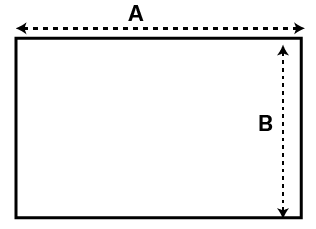• Area of a square $\text{= side }\!\!\times\!\!\text{ side }\!\!~\!\!\text{ }$